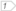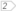#Boost C++ Libraries

...one of the most highly regarded and expertly designed C++ library projects in the world.

## Tutorial

Generating integers in a range
Generating integers with different probabilities

### Generating integers in a range

For the source of this example see die.cpp. First we include the headers we need for `mt19937` and `uniform_int_distribution`.

```#include <boost/random/mersenne_twister.hpp>
#include <boost/random/uniform_int_distribution.hpp>```

We use `mt19937` with the default seed as a source of randomness. The numbers produced will be the same every time the program is run. One common method to change this is to seed with the current time (`std::time(0)` defined in ctime).

`boost::random::mt19937 gen;`

NoteWe are using a global generator object here. This is important because we don't want to create a new pseudo-random number generator at every call

Now we can define a function that simulates an ordinary six-sided die.

```int roll_die() {boost::random::uniform_int_distribution<> dist(1, 6);return dist(gen);
}
```

`mt19937` produces integers in the range [0, 232-1]. However, we want numbers in the range [1, 6]. The distribution `uniform_int_distribution` performs this transformation.

WarningContrary to common C++ usage `uniform_int_distribution` does not take a half-open range. Instead it takes a closed range. Given the parameters 1 and 6, `uniform_int_distribution` can can produce any of the values 1, 2, 3, 4, 5, or 6.

A distribution is a function object. We generate a random number by calling `dist` with the generator.

### Generating integers with different probabilities

For the source of this example see weighted_die.cpp.

```#include <boost/random/mersenne_twister.hpp>
#include <boost/random/discrete_distribution.hpp>

boost::mt19937 gen;```

This time, instead of a fair die, the probability of rolling a 1 is 50% (!). The other five faces are all equally likely.

`discrete_distribution` works nicely here by allowing us to assign weights to each of the possible outcomes.

TipIf your compiler supports `std::initializer_list`, you can initialize `discrete_distribution` directly with the weights.

```double probabilities[] = {
0.5, 0.1, 0.1, 0.1, 0.1, 0.1
};
boost::random::discrete_distribution<> dist(probabilities);```

Now define a function that simulates rolling this die.

```int roll_weighted_die() {return dist(gen) + 1;
}

```

 Add 1 to make sure that the result is in the range [1,6] instead of [0,5].

For the source of this example see password.cpp.

This example demonstrates generating a random 8 character password.

```#include <boost/random/random_device.hpp>
#include <boost/random/uniform_int_distribution.hpp>

int main() {std::string chars(
"abcdefghijklmnopqrstuvwxyz"
"ABCDEFGHIJKLMNOPQRSTUVWXYZ"
"1234567890"
"!@#\$%^&*()"
"`~-_=+[{]{\\|;:'\",<.>/? ");boost::random::random_device rng;boost::random::uniform_int_distribution<> index_dist(0, chars.size() - 1);
for(int i = 0; i < 8; ++i) {
std::cout << chars[index_dist(rng)];
}
std::cout << std::endl;
}

```

 We first define the characters that we're going to allow. This is pretty much just the characters on a standard keyboard. We use `random_device` as a source of entropy, since we want passwords that are not predictable. Finally we select 8 random characters from the string and print them to cout.